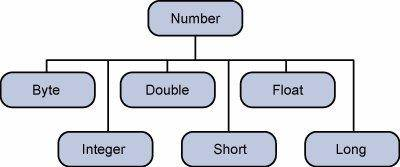# Java Number类

2022-01-25 12:02 更新

``````public static void main(String[] args) {
int num1 = 1;
//将基本数据类型装箱成对象包装类型
Integer num2 = num1;
Integer num3 = 3;
//将对象数据类拆箱
int num4 = num3;
}
``````

Number 类是 java.lang 包下的一个抽象类，提供了将包装类型拆箱成基本类型的方法，所有基本类型（数据类型）的包装类型都继承了该抽象类，并且是final声明不可继承改变；

``````package java.lang;

public abstract class Number implements java.io.Serializable {

public abstract int intValue();

public abstract long longValue();

public abstract float floatValue();

public abstract double doubleValue();

public byte byteValue() {
return (byte)intValue();
}

public short shortValue() {
return (short)intValue();
}

private static final long serialVersionUID = -8742448824652078965L;
}``````

Boolean boolean
Byte byte
Short short
Integer int
Long long
Character char
Float float
Double double``````public class Test{

public static void main(String args[]){
Integer x = 5; // boxes int to an Integer object
x =  x + 10;   // unboxes the Integer to a int
System.out.println(x);
}
}``````

``15``

# Java Math类

Java 的 Math 包含了用于执行基本数学运算的属性和方法，如初等指数、对数、平方根和三角函数。

Math 的方法都被定义为 static 形式，通过 Math 类可以在主函数中直接调用。

``````public class Test {
public static void main (String []args)
{
System.out.println("90 度的正弦值：" + Math.sin(Math.PI/2));
System.out.println("0度的余弦值：" + Math.cos(0));
System.out.println("60度的正切值：" + Math.tan(Math.PI/3));
System.out.println("1的反正切值： " + Math.atan(1));
System.out.println("π/2的角度值：" + Math.toDegrees(Math.PI/2));
System.out.println(Math.PI);
}
}``````

``````90 度的正弦值：1.0
0度的余弦值：1.0
60度的正切值：1.7320508075688767
1的反正切值： 0.7853981633974483
π/2的角度值：90.0
3.141592653589793``````

## Number & Math 类方法

1 xxxValue()

2 compareTo()

3 equals()

4 valueOf()

5 toString()

6 parseInt()

7 abs()

8 ceil()

9 floor()

10 rint()

11 round()

12 min()

13 max()

14 exp()

15 log()

16 pow()

17 sqrt()

18 sin()

19 cos()

20 tan()

21 asin()

22 acos()

23 atan()

24 atan2()

25 toDegrees()

27 random()

App下载